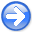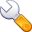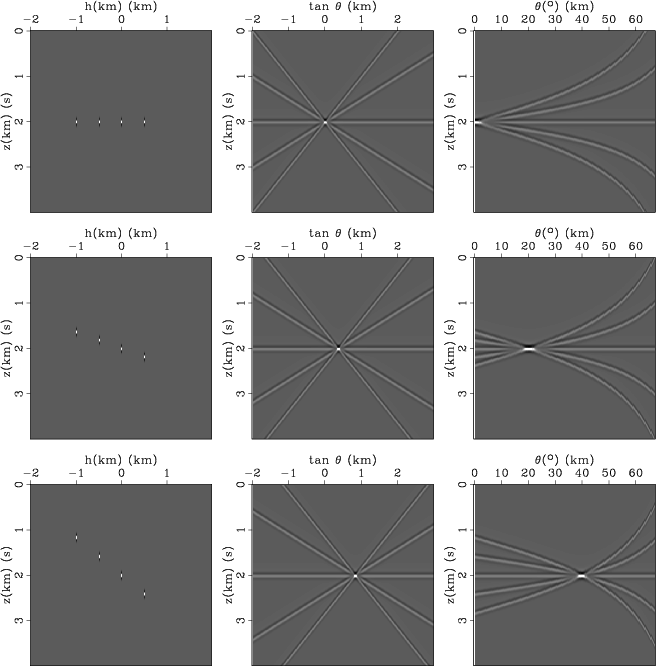Time-shift imaging condition in seismic migrationNext: Conclusions Up: Time-shift imaging condition in Previous: Examples

# Discussion

As discussed in one of the preceding sections, time-shift gathers consist of linear events with slopes corresponding to the local migration velocity. In contrast, space-shift gathers consist of events focused at. Those events can be mapped to the angle-domain using transformations (20) and (23), respectively.

In order to understand the angle-domain mapping, we consider a simple synthetic in which we model common-image gathers corresponding to incidence at a particular angle. The experiment is depicted in Figure 15 for time-shift imaging, and in Figure 16 for space-shift imaging. For this experiment, the sampling parameters are the following:km,km, ands.

A reflection event at a single angle of incidence maps in common-image gathers as a line of a given slope. The left panels in Figures 15 and 16 showcases, corresponding to angles of,and. Since we want to analyze how such events map to angle, we subsample each line toselected samples lining-up at the correct slope.

The middle panels in Figures 15 and 16 show the data in the left panels after slant-stacking inorpanels, respectively. Each individual sample from the common-image gathers maps in a line of a different slope intersecting in a point. For example, normal incidence in a time-shift gather maps at the migration velocitykm/s (Figure 15 top row, middle panel), and normal incidence in a space-shift gather maps at slant-stack parameter.

The right panels in Figures 15 and 16 show the data from the middle panels after mapping to angle using equations (23) and (20), respectively. All lines from the slant-stack panels map into curves that intersect at the angle of incidence.

We note that all curves for the time-shift angle-gathers have zero curvature at normal incidence. Therefore, the resolution of the time-shift mapping around normal incidence is lower than the corresponding space-shift resolution. However, the storage and computational cost of time-shift imaging is smaller than the cost of equivalent space-shift imaging. The choice of the appropriate imaging condition depends on the imaging objective and on the trade-off between the cost and the desired resolution.ttest
Figure 15.
Image-gather formation using time-shift imaging. Each row depicts an event at(top),(middle), and(bottom). Three columns correspond to subsampled time-shift gathers (left), slant-stacked gathers (middle), and angle-gathers (right).htest
Figure 16.
Image-gather formation using space-shift imaging. Each row depicts an event at(top),(middle), and(bottom). Three columns correspond to subsampled space-shift gathers (left), slant-stacked gathers (middle), and angle-gathers (right).Time-shift imaging condition in seismic migrationNext: Conclusions Up: Time-shift imaging condition in Previous: Examples

2007-04-08